Test: Fractions - 2

# Test: Fractions - 2

Test Description

## 10 Questions MCQ Test Mathematics for Class 4 | Test: Fractions - 2

Test: Fractions - 2 for Class 4 2023 is part of Mathematics for Class 4 preparation. The Test: Fractions - 2 questions and answers have been prepared according to the Class 4 exam syllabus.The Test: Fractions - 2 MCQs are made for Class 4 2023 Exam. Find important definitions, questions, notes, meanings, examples, exercises, MCQs and online tests for Test: Fractions - 2 below.
Solutions of Test: Fractions - 2 questions in English are available as part of our Mathematics for Class 4 for Class 4 & Test: Fractions - 2 solutions in Hindi for Mathematics for Class 4 course. Download more important topics, notes, lectures and mock test series for Class 4 Exam by signing up for free. Attempt Test: Fractions - 2 | 10 questions in 15 minutes | Mock test for Class 4 preparation | Free important questions MCQ to study Mathematics for Class 4 for Class 4 Exam | Download free PDF with solutions
 1 Crore+ students have signed up on EduRev. Have you?
Test: Fractions - 2 - Question 1

### Which of the fraction is least?

Detailed Solution for Test: Fractions - 2 - Question 1

Calculations:
24/25 = 0.96
10/11 = 0.90
99/100 = 0.99
68/69 = 0.98
∴ 10/11 is the least fraction.

Test: Fractions - 2 - Question 2

### Which of the following is true?

Detailed Solution for Test: Fractions - 2 - Question 2

Concept Used:
a/b and c/d are two numbers,
then (a × d) and (b × c) are need to be compared.

Calculation:
We have, 9/16 and 13/24
⇒ 9 × 24 = 216
⇒ 13 × 16 = 208
⇒ 216 > 208
⇒ 9/16 > 13/24.
∴ Option 3 is correct.

Test: Fractions - 2 - Question 3

### If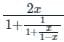= 1, then the value of x is:

Detailed Solution for Test: Fractions - 2 - Question 3= 1
⇒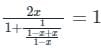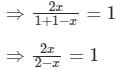⇒ 2x = 2 - x
⇒ 3x = 2
⇒ x = 2/3
∴ The value of x is 2/3
The correct option is 3 i.e. 2/3

Test: Fractions - 2 - Question 4

If (2x + 3y)/(3x - 4y) = 11/7,  then the value of x/y is ________

Detailed Solution for Test: Fractions - 2 - Question 4

(2x + 3y)/(3x - 4y) = 11/7
⇒ 14x + 21y = 33x - 44y
⇒ 65y = 19x
∴ x/y = 65/19

Test: Fractions - 2 - Question 5

Arrange the fractions 3/4, 5/12, 11/18, 17/24 in ascending order

Detailed Solution for Test: Fractions - 2 - Question 5

Given:
Our given fraction is 3/4, 5/12, 11/18, 17/24
Caoncept used:
Make denominator of each fraction same,
Calculation:
3/4 = (3 × 18) / (4 × 18) = 54/72
5/12 = (5 × 6) / (12 × 6) = 30/72
11/18 = (11 × 4) / (18 × 4) = 44/72
17/24 = (17 × 3) / (24 × 3) = 51/72
∴ Ascending order of given franctions is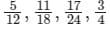Test: Fractions - 2 - Question 6

If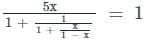, then find the value of 'x'.

Detailed Solution for Test: Fractions - 2 - Question 6

Given:Calculation:
⇒⇒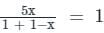⇒ 5x/(2 – x) = 1
⇒ 5x = 2 – x
⇒ 6x = 2
⇒ x = 2/6
∴ The required value of x is 1/3.

Test: Fractions - 2 - Question 7

In a group of students, 1/5 are aged below 8 years. Of the remaining 2/5 are above 8 years. What fraction of the students are exactly 8 years of age?

Detailed Solution for Test: Fractions - 2 - Question 7

Let the total number of students in the group be 1

Number of students who are below age 8 = 1 × 1/5 = 1/5

Remaining students = 1 – 1/5 = 4/5

Number of students who are above age 8 = 4/5 × 2/5 = 8/25

Number of students who are exactly 8 years old = 1 – 1/5 – 8/25 = 12/25

Test: Fractions - 2 - Question 8

What will come in place of question mark '?' in the following question?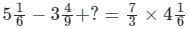Detailed Solution for Test: Fractions - 2 - Question 8

Calculations:
Given expression is,
⇒⇒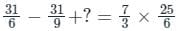⇒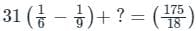⇒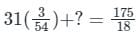⇒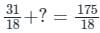⇒ ? = 144/18
⇒ ? = 8

Test: Fractions - 2 - Question 9

Directions: What will come in place of the question mark (?) in the following questions?
Q. ? = {(2.5)3 + (1.5)3}/{(2.5)3 – (1.5)3}

Detailed Solution for Test: Fractions - 2 - Question 9

Given:
{(2.5)3 + (1.5)3}/{(2.5)3 – (1.5)3}
Calculation:
⇒ ? = {(2.5)3 + (1.5)3}/{(2.5)3 – (1.5)3}
⇒ ? = (15.625 + 3.375)/(15.625 - 3.375)
⇒ ? = 19/12.25
⇒ ? = 1900/1225 = 76/49

Test: Fractions - 2 - Question 10

If the fractions 7/13, 2/3, 4/11, 5/9 are arranged in ascending order, then the correct sequence is ?

Detailed Solution for Test: Fractions - 2 - Question 10

(7/13) = 0.538
(2/3) = 0.666
(4/11) = 0.3636
(5/9) = 0.5555
Out of 2/3, 7/13, 4/11, 5/9
2/3 is the largest number followed by 5/9 then 7/13 and the smallest is 4/11.
∴ The ascending order will be 4/11, 7/13, 5/9, 2/3.

## Mathematics for Class 4

17 videos|68 docs|32 tests
 Use Code STAYHOME200 and get INR 200 additional OFF Use Coupon Code
Information about Test: Fractions - 2 Page
In this test you can find the Exam questions for Test: Fractions - 2 solved & explained in the simplest way possible. Besides giving Questions and answers for Test: Fractions - 2, EduRev gives you an ample number of Online tests for practice

## Mathematics for Class 4

17 videos|68 docs|32 tests